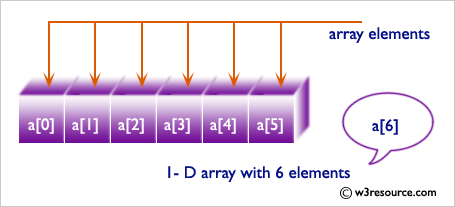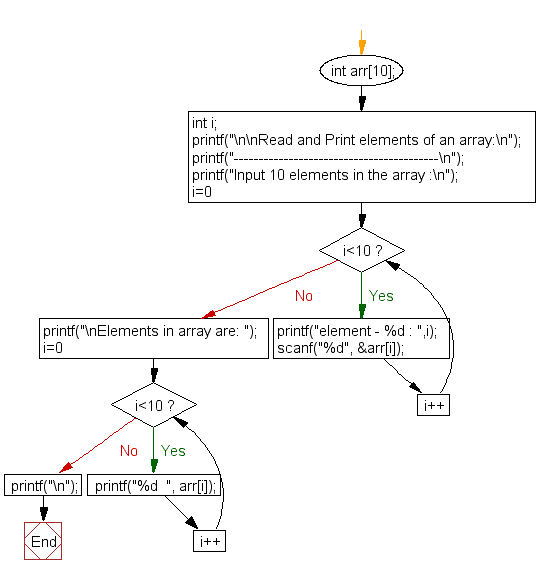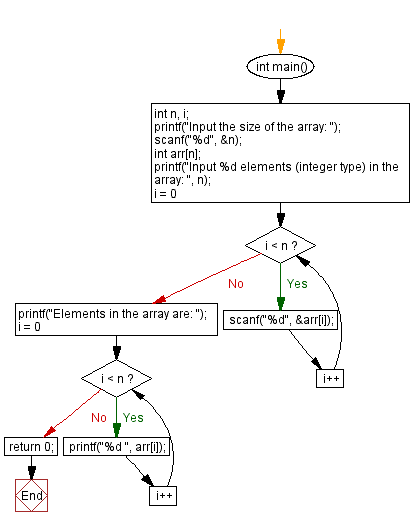﻿ C Program: Read and Print elements of an array - w3resource

# C Exercises: Read and Print elements of an array

## C Array: Exercise-1 with Solution

Write a program in C to store elements in an array and print them.

Visual Presentation:Sample Solution:

C Code:

``````#include <stdio.h>
// Main function
int main()
{
int arr;  // Declare an array of size 10 to store integer values
int i;
// Print a message to prompt the user for input
printf("\n\nRead and Print elements of an array:\n");
printf("-----------------------------------------\n");
// Prompt the user to input 10 elements into the array
printf("Input 10 elements in the array :\n");
for(i=0; i<10; i++)
{
printf("element - %d : ",i);  // Prompt the user to input the i-th element
scanf("%d", &arr[i]);  // Read the input and store it in the array
}
// Display the elements in the array
printf("\nElements in array are: ");
for(i=0; i<10; i++)
{
printf("%d  ", arr[i]);  // Print each element in the array
}
printf("\n");
return 0;
}
```
```

Sample Output:

```Read and Print elements of an array:
-----------------------------------------
Input 10 elements in the array :
element - 0 : 1
element - 1 : 1
element - 2 : 2
element - 3 : 3
element - 4 : 4
element - 5 : 5
element - 6 : 6
element - 7 : 7
element - 8 : 8
element - 9 : 9

Elements in array are: 1  1  2  3  4  5  6  7  8  9
```

Explanation:

```   printf("Input 10 elements in the array :\n");
for(i=0; i<10; i++)
{
printf("element - %d : ",i);
scanf("%d", &arr[i]);
}

printf("\nElements in array are: ");
for(i=0; i<10; i++)
{
printf("%d  ", arr[i]);
}
```

The above C code prompts the user to input 10 integers into an array named arr, and then prints out the elements of the array.

The first printf statement asks the user to input 10 elements into the array, and the for loop runs from i=0 to i<10, prompting the user to enter each element using scanf, and storing each input in the corresponding index of the array arr[i].

The second printf statement then prints out the contents of the array using another for loop, which iterates over the elements of arr and prints each element out using printf.

Flowchart:How to read and print elements of a C array of any size?

C Code:

``````#include <stdio.h>
// Main function
int main() {
int n, i;
// Prompt the user to input the size of the array
printf("Input the size of the array: ");
scanf("%d", &n);

// Declare an array of size n to store integer values
int arr[n];
// Prompt the user to input n elements into the array
printf("Input %d elements (integer type) in the array: ", n);
for (i = 0; i < n; i++) {
scanf("%d", &arr[i]); // Read the input and store it in the array
}
// Display the elements in the array
printf("Elements in the array are: ");
for (i = 0; i < n; i++) {
printf("%d ", arr[i]); // Print each element in the array
}
return 0; // Indicate successful execution of the program
}
``````

Sample Output:

```Input the size of the array: 5
Input 5 elements (integer type) in the array: 1
3
5
7
9
Elements in the array are: 1 3 5 7 9
```

Explanation:

The above program first prompts the user to input the array size and stores it in the integer variable n. It then declares an integer array nums of size n and an integer variable i. The program then prompts the user to input n number of elements into the array through a for loop that runs from i=0 to i<n. Finally, the program prints the elements of the array using another for loop that runs from i=0 to i<n and uses the printf() function to print each element of the array.

Flowchart:C Programming Code Editor:

What is the difficulty level of this exercise?

Test your Programming skills with w3resource's quiz.

﻿